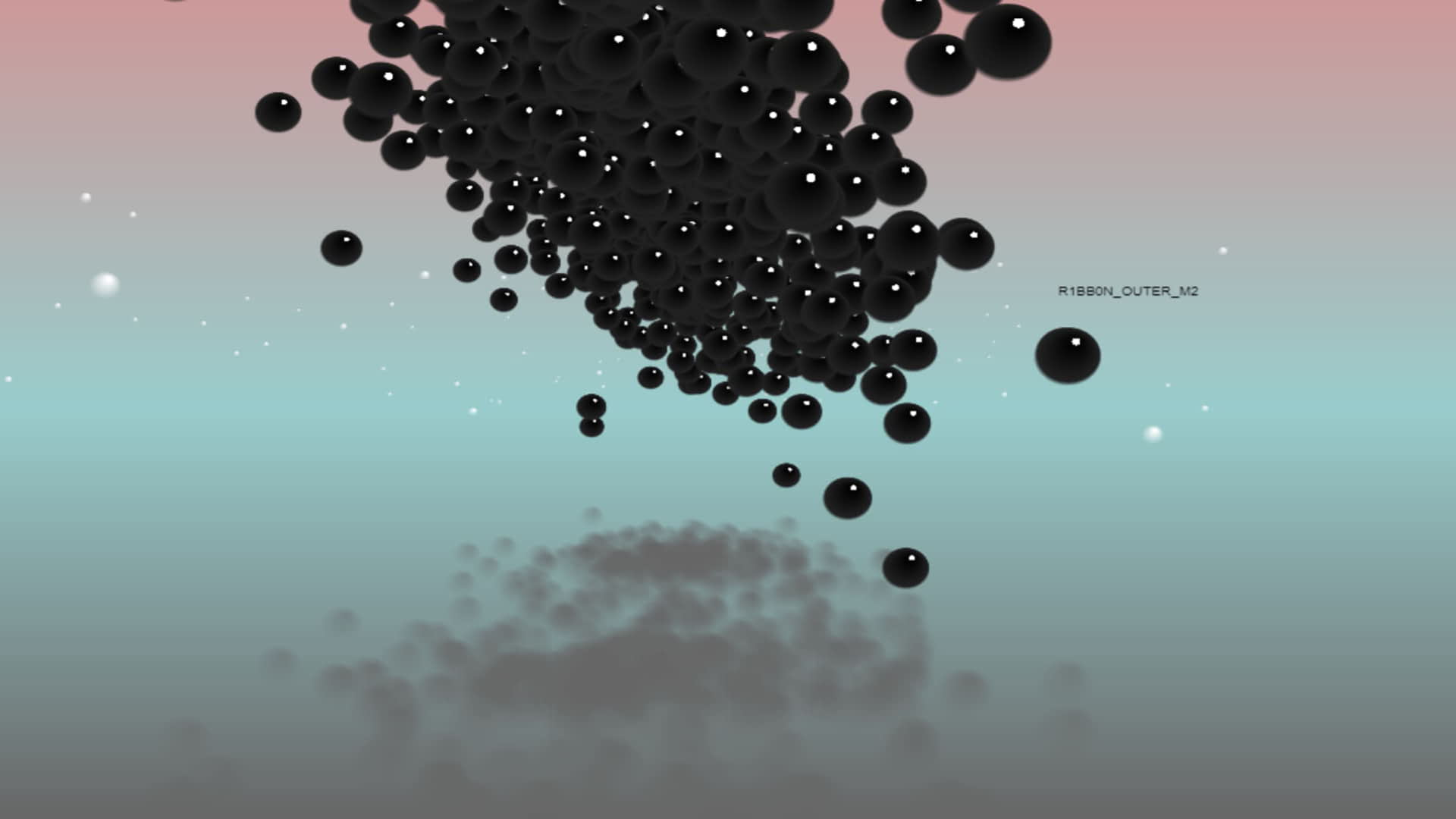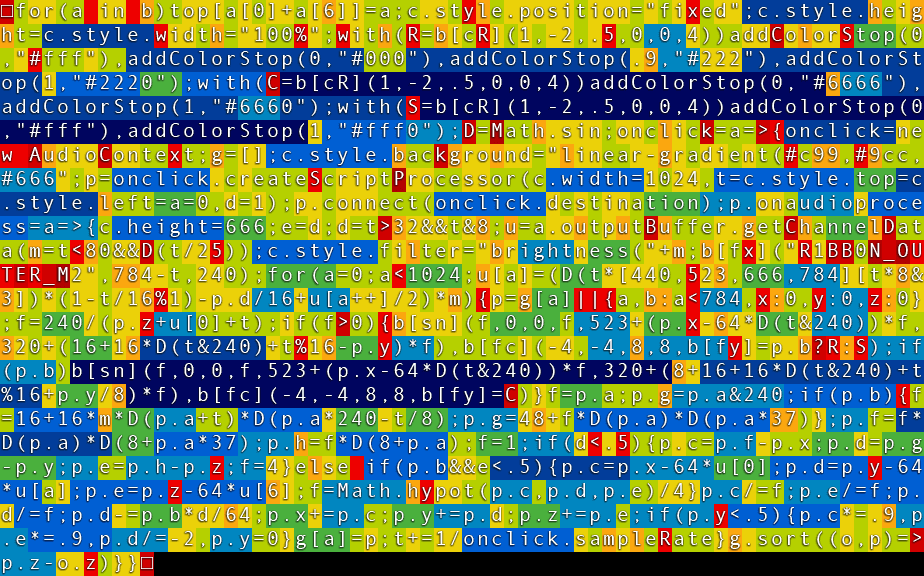# OUTER_M2

715 words ~ 3-7 minsMathieu 'p01' Henri on August 3rd, 2019

# OUTER_M2

OUTER_M2: EXPLODING NEUTRINOS, a smashing JavaScript demo for the 1024 bytes demo competition at the Assembly 2019.The Assembly is a 27 years old demoscene event with the most anticipated 1kb, 4kb, 64kb and 256mb demo competitions. I entered the 1kb competition several times and had the honor to get 1st with BLCK4777 and VOLTRA 2nd place with ASTRA. Let's hope that OUTER_M2 holds up.

## Exploding neutrinos in 1021 bytes

For OUTER_M2, the goal was to get a more modern look and some physics simulation.

The compressed version OUTER_M2 is 1021 bytes big. Just click the page after a short while to start the demo.

Here is a video capture.

## Technical breakdown

The rules of the Assembly, and other demoscene competitions, is that the entry must be released as a single file, ready to run from file system. For the 1kb competition, the size limit is 1024 bytes. Since the entry must run from file system we can not use Gzip. To get the most we must somehow compress our entry and fit the compressed data and the decompressor code into 1024 bytes.

### Minified code

The minified code of OUTER_M2 is 1527 bytes long.

``for(a in b)top[a+a]=a;c.style.position="fixed";c.style.height=c.style.width="100%";with(R=b[cR](1,-2,.5,0,0,4))addColorStop(0,"#fff"),addColorStop(0,"#000"),addColorStop(.9,"#222"),addColorStop(1,"#2220");with(C=b[cR](1,-2,.5,0,0,4))addColorStop(0,"#6666"),addColorStop(1,"#6660");with(S=b[cR](1,-2,.5,0,0,4))addColorStop(0,"#fff"),addColorStop(1,"#fff0");D=Math.sin;onclick=a=>{onclick=new AudioContext;g=[];c.style.background="linear-gradient(#c99,#9cc,#666";p=onclick.createScriptProcessor(c.width=1024,t=c.style.top=c.style.left=a=0,d=1);p.connect(onclick.destination);p.onaudioprocess=a=>{c.height=666;e=d;d=t>32&&t&8;u=a.outputBuffer.getChannelData(m=t<80&&D(t/25));c.style.filter="brightness("+m;b[fx]("R1BB0N_OUTER_M2",784-t,240);for(a=0;a<1024;u[a]=(D(t*[440,523,666,784][t*8&3])*(1-t/16%1)-p.d/16+u[a++]/2)*m){p=g[a]||{a,b:a<784,x:0,y:0,z:0};f=240/(p.z+u+t);if(f>0){b[sn](f,0,0,f,523+(p.x-64*D(t&240))*f,320+(16+16*D(t&240)+t%16-p.y)*f),b[fc](-4,-4,8,8,b[fy]=p.b?R:S);if(p.b)b[sn](f,0,0,f,523+(p.x-64*D(t&240))*f,320+(8+16+16*D(t&240)+t%16+p.y/8)*f),b[fc](-4,-4,8,8,b[fy]=C)}f=p.a;p.g=p.a&240;if(p.b){f=16+16*m*D(p.a+t)*D(p.a*240-t/8);p.g=48+f*D(p.a)*D(p.a*37)};p.f=f*D(p.a)*D(8+p.a*37);p.h=f*D(8+p.a);f=1;if(d<.5){p.c=p.f-p.x;p.d=p.g-p.y;p.e=p.h-p.z;f=4}else if(p.b&&e<.5){p.c=p.x-64*u;p.d=p.y-64*u[a];p.e=p.z-64*u;f=Math.hypot(p.c,p.d,p.e)/4}p.c/=f;p.e/=f;p.d/=f;p.d-=p.b*d/64;p.x+=p.c;p.y+=p.d;p.z+=p.e;if(p.y<.5){p.c*=.9,p.e*=.9,p.d/=-2,p.y=0}g[a]=p;t+=1/onclick.sampleRate}g.sort((o,p)=>p.z-o.z)}}``

It assumes the following setup which takes another 59 bytes + some to load and run the minified code above.

``<canvas id="c"></canvas><script>b=c.getContext`2d`</script>``

All in all, this about 1586 bytes of minified code that we'll have to compress down to 1024 bytes of self extracting code.

### Compression & PNG bootstrapping

The final file, OUTER_M2.packed.html, is a polyglot file which is both a PNG image and an HTML page with a bit Javascript that loads the file in an `IMG` element, draws the pixels in a `CANVAS` and treats them as the ASCII value of a long string of character which is finally evaluated. This way of bootstrapping a PNG image allows to ship a single file and to benefit from the compression ratio of PNG and ZLIB, and all the tooling that comes with it.

The code of the PNG bootstrapper takes 157 bytes.

``<canvas id=c><img onload=b=c.getContext`2d`;for(p=e='';t=b.getImageData(0,0,1,!b.drawImage(this,p--,0)).data;)e+=String.fromCharCode(t);(1,eval)(e) src=#>``

This means the PNG image must be no bigger than `1024 - 157 = 867` bytes after removing the IEND chunk. Taking into account the PNG header and various chunk headers, this leave a mere `822 bytes` of DEFLATE stream for the code of the demo.

In other words: there really isn't much space to do something cool.Above is a thermal view of the DEFLATE stream for OUTER_M2, where the dark/cold colors represent characters that compressed the most, and warm colors represent characters that did not.

### Audio setup

For the Audio setup, I use the deprecated but very useful `ScriptProcessorNode` from the Web Audio API, and update the 1024 bytes of channel data when the `audioprocess` event fires every ~42ms

### Music

Here is the whole code for the music.

``u[a]=(D(t*[440,523,666,784][t*8&3])*(1-t/16%1)-p.d/16+u[a++]/2)*m``

It is made of 4 notes arpeggio with a sinus oscillator ( `D(t[440,523,666,784][t8&3])` ) with the volume going every 16s ( `(1-t/16%1)` ) a basic echo ( `+u[a++]/2` ) a master volume ( `(...)*m ` ) and I added the vertical velocity of the particles ( `-p.d/16` ) to the mix to glitch the audio in sync with the particles ;)